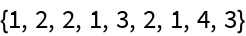#Function Repository Resource:

# StandardOrderFromIndex

Get the standard ordering of the desired index

Contributed by: Ed Pegg Jr
 ResourceFunction["StandardOrderFromIndex"][index,len] gives the standard ordering of length len with position index.

## Details and Options

Integers greater than zero in standard order start with 1, then can never be more than 1 higher than all previous integers.
The index is acquired by first noting the positions of the highest numbers to make a binary number.
The table below illustrates the process for determining an index for a standard order:
 {1,2,2,1,3,2,1,4,3} integers >0 in standard order start with 1, then can never be more than 1 higher than all previous integers. {1,2,0,0,3,0,0,4,0} some of the digits are new highest digits. {0,0,2,1,0,2,1,0,3} other digits acquire a mixed radix based on the previous highest digit. {1,0,0,1,0,0,1,0} without the initial 1, the sequence of highest digits mapped to 1 values makes a binary number B. 223241 any binary number with 1's→{2,…,n} and sequential zeros acting as powers gives a Bell index. BellB [ n ] equals the sum of Bell indices of length n-1 binary numbers. {146,86}→5867 the total of the first B Bell indices plus the mixed radix value gives the index.
Standard orderings are sorted based on binary index B.
The result follows the "standard order" described in the OEIS.

## Examples

### Basic Examples

Return the index of a permutation:

 In:=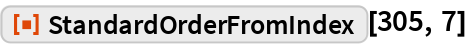Out=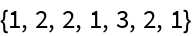The 52 standard orders of length 5:

 In:=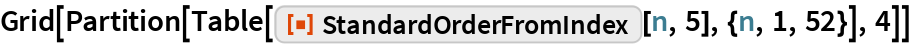Out=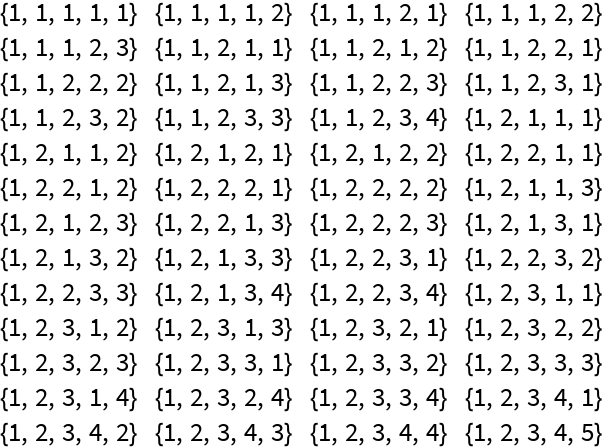### Scope

There are 10 billion standard orderings of length 16:

 In:=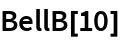Out=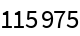Give the length 16 standard ordering with position 5555555555:

 In:=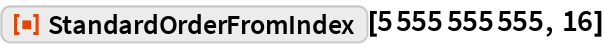Out=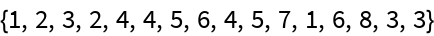Find the standard order with a given length and index:

 In:=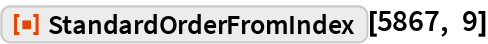Out=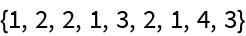### Properties and Relations

 In:=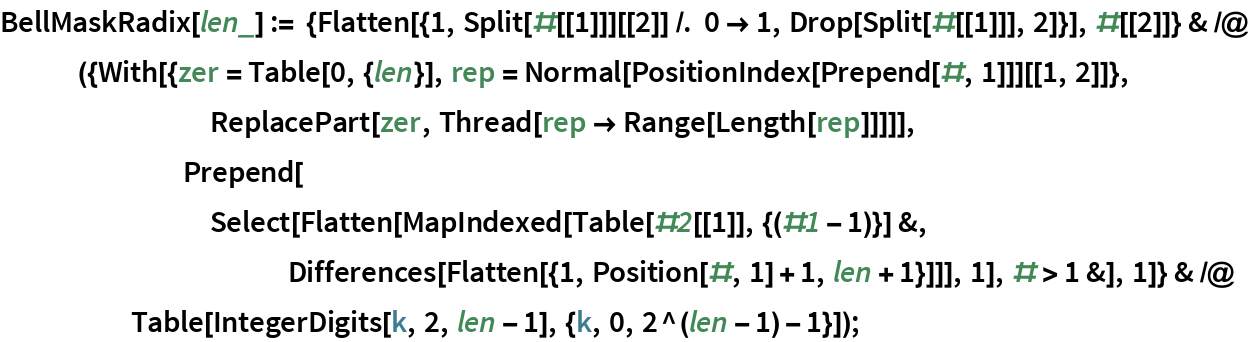This provides a method for going from ordering to index:

 In:=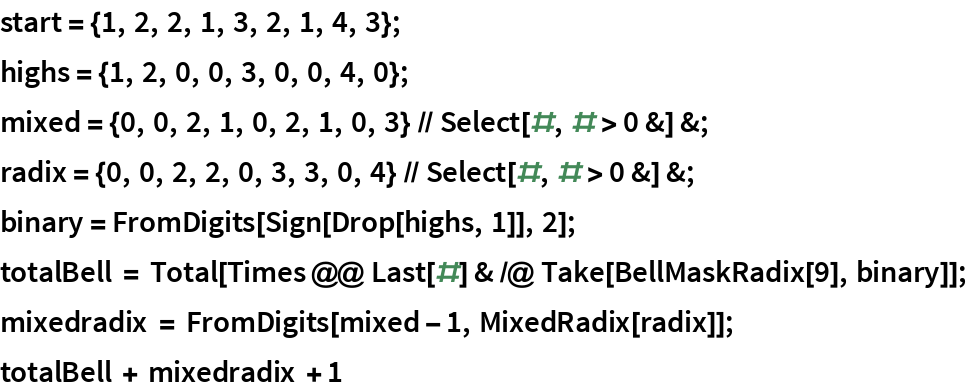Out=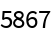This matches the result from StandardOrderFromIndex:

 In:=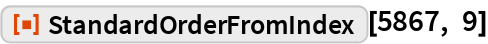Out=